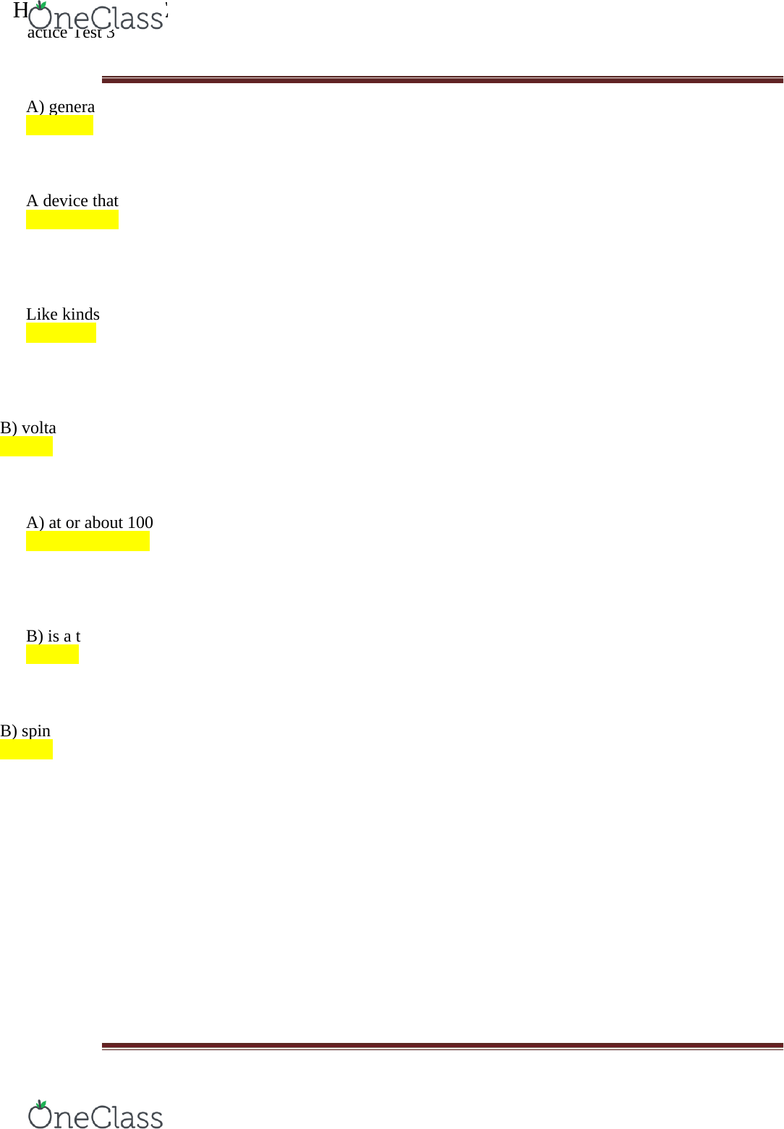# PSC 2121 Lecture Notes - Lecture 5: Transverse Wave, Atomic Nucleus, Einsteinium

31 views6 pages
School
Course
Page:
of 6PHYSICAL SCIENCE PSC2121
Practice Test 3
November 3, 2015
1. A device that transforms electrical energy to mechanical energy is a
A) generator.
B) motor.
C) transformer.
D) magnet.
E) none of the above
2. A device that transforms mechanical energy to electrical energy is a
A) generator.
B) motor.
C) transformer.
D) magnet.
E) none of the above
3. Like kinds of magnetic poles repel while unlike kinds of magnetic poles
A) attract.
B) repel also.
C) may attract or repel.
4. Changing the magnetic field intensity in a closed loop of wires induces
A) current.
B) voltage.
C) both
D) neither
5. Magnet A has twice the magnetic field strength of magnet B and pulls on magnet B with
a force of 200 N. The amount of force that magnet A exerts on magnet B is
A) at or about 100 N.
B) exactly 200 N.
C) more than 200 N.
D) not enough information
6. Every spinning electron is
A) electrically charged.
B) is a tiny magnet.
C) both
D) none of the above
7. Magnetism is due to the motion of electrons as they
A) move around the nucleus.
B) spin on their axes.
C) both
D) neither
Page 1
find more resources at oneclass.com
find more resources at oneclass.com
PHYSICAL SCIENCE PSC2121
8. If you double the frequency of a vibrating object, its period
A) doubles.
B) is half.
C) is one quarter.
9. Which of the following is not a transverse wave?
A) sound
B) light
C) radio
D) all of the above
E) none of the above
10. Compressions and rarefactions are characteristic of
A) longitudinal waves.
B) transverse waves.
C) both
D) neither
11. The range of human hearing is about
A) 10 Hz - 10,000 Hz.
B) 20 Hz - 20,000 Hz.
C) 40 Hz - 40,000 Hz.
D) all of the above, depending on the person
12. A wave oscillates up and down two complete cycles each 1 s. If the wave travels an
average distance of 6 m in 1 s, its wavelength is
A) 0.5 m.
B) 1 m. 2/1(# of cycles/time) = 2 …. 6/1 (v=lambda/time)=6 …. 6/2= 3m
C) 2 m.
D) 3 m.
E) 6 m.
13. During a single period, the distance traveled by a wave is
A) one-half wavelength.
B) one wavelength.
C) two wavelengths.
14. Which of these has the longest wavelength?
A) radio waves
B) microwaves
C) visible light
D) gamma rays
15. If an electric charge is rapidly shaken up and down,
A) sound is emitted.
B) light is emitted.
C) electron excitation occurs.
Page 2
find more resources at oneclass.com
find more resources at oneclass.com
PHYSICAL SCIENCE PSC2121
D) an electromagnetic wave is produced.
16. Relative to ultraviolet waves, the wavelength of gamma rays is
A) shorter.
B) longer.
C) the same.
17. A skipper on a boat notices wave crests passing the anchor chain every 5 s. The skipper
estimates the distance between crests is 15 m. What is the speed of the water waves?
A) 3 m/s
B) 5 m/s
C) 15 m/s
D) not enough information
18. Light will not pass through a pair of Polaroids when their axes are
A) parallel.
B) perpendicular.
C) 45° to each other.
D) all of the above
E) none of the above
19. Which contributes more to an atom's mass: electrons or protons? Which contributes more
to an atom's size?
A) Protons contribute more to an atom's mass while electrons contribute more to its size.
B) Electrons contribute more to an atom's mass while protons contribute more to its size.
C) Protons contribute more to both the mass and size of an atom.
D) Electrons contribute more to both the mass and size of an atom.
20. If an atom has 55 electrons, 78 neutrons, and 55 protons, what is its approximate atomic
mass? What is the name of this element?
A) atomic mass, 133 amu; Caesium (atomic mass= proton+ neutrons)
B) atomic mass, 99 amu; Technetium
C) atomic mass, 99 amu; Radon
D) atomic mass 142 amu; Einsteinium
21. If two protons and two neutrons are removed from the nucleus of a gold atom, a nucleus
of which element remains?
A) iridium-194
B) iridium-192
C) gold-192
D) gold-194
22. If a neutral element has the following chemical symbol, how many electrons does it
have?
39K
19
Page 3
find more resources at oneclass.com
find more resources at oneclass.com